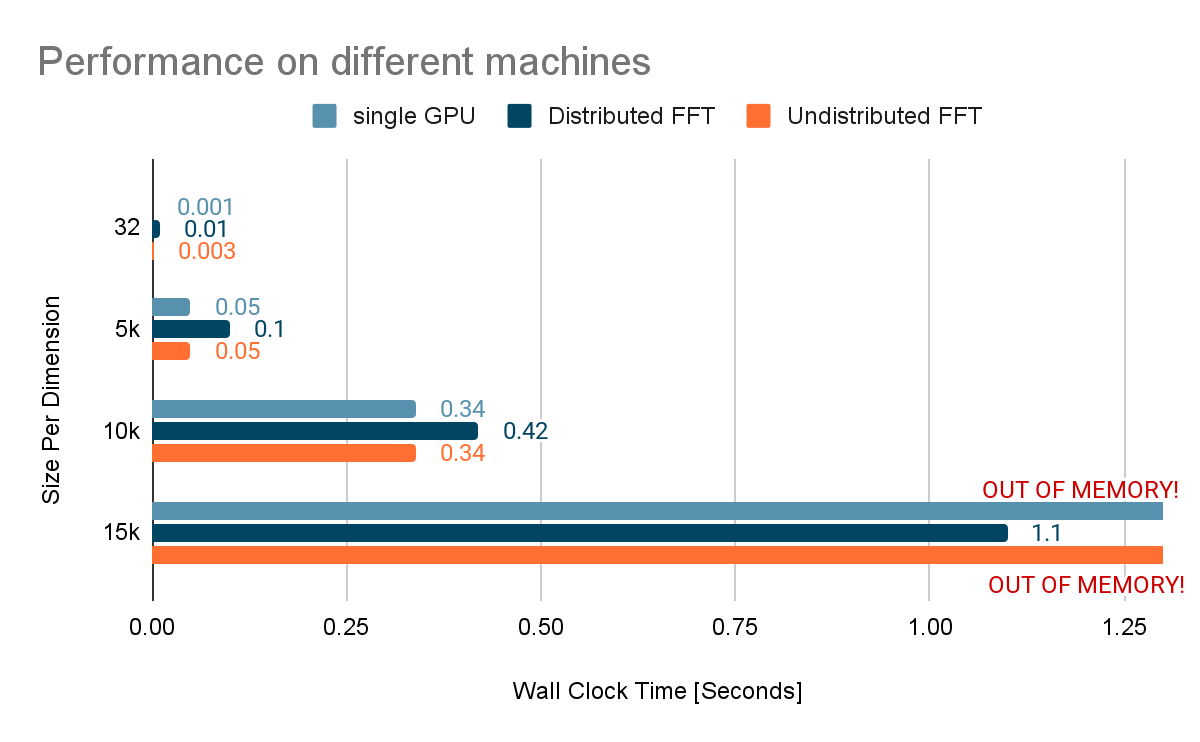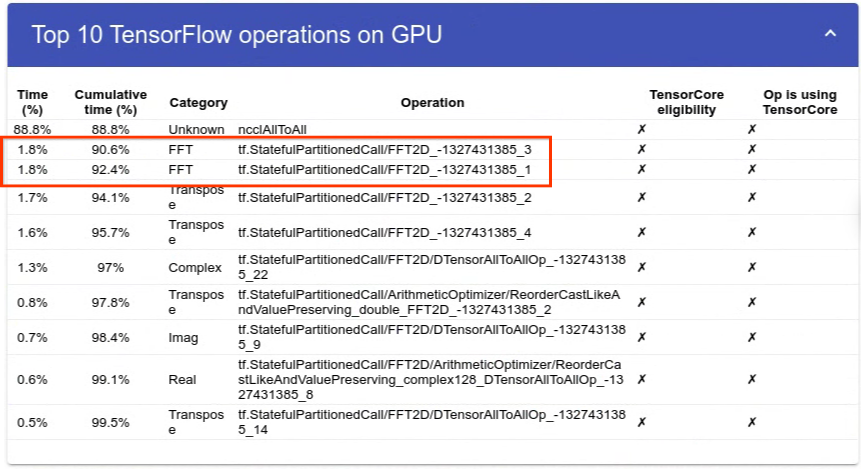TensorFlow Core ·Distributed Fast Fourier Transform in TensorFlow
August 24, 2023Posted by Ruijiao Sun, Google Intern - DTensor teamFast Fourier Transform is an important method of signal processing, which is commonly used in a number of ways, including speeding up convolutions, extracting features, and regularizing models. Distributed Fast Fourier Transform (Distributed FFT) offers a way to compute Fourier Transforms in models that work with image-like datasets that are too large to fit into the memory of a single accelerator device. In a previous Google Research Paper, “Large-Scale Discrete Fourier Transform on TPUs” by Tianjian Lu, a Distributed FFT algorithm was implemented for TensorFlow v1 as a library. This work presents the newly added native support in TensorFlow v2 for Distributed FFT, through the new TensorFlow distribution API, DTensor.

DTensor is an extension to TensorFlow for synchronous distributed computing. It distributes the program and tensors through a procedure called Single program, multiple data (SPMD) extension. DTensor offers an uniform API for traditional data and model parallelism patterns used widely in Machine Learning.

## Example Usage

The API interface for distributed FFT is the same as the original FFT in TensorFlow. Users just need to pass a sharded tensor as an input to the existing FFT ops in TensorFlow, such as tf.signal.fft2d. The output of a distributed FFT becomes sharded too.

 ```import TensorFlow as tf from TensorFlow.experimental import dtensor # Set up devices device_type = dtensor.preferred_device_type() if device_type == 'CPU': cpu = tf.config.list_physical_devices(device_type) tf.config.set_logical_device_configuration(cpu, [tf.config.LogicalDeviceConfiguration()] * 8) if device_type == 'GPU': gpu = tf.config.list_physical_devices(device_type) tf.config.set_logical_device_configuration(gpu, [tf.config.LogicalDeviceConfiguration(memory_limit=1000)] * 8) dtensor.initialize_accelerator_system() # Create a mesh mesh = dtensor.create_distributed_mesh(mesh_dims=[('x', 1), ('y', 2), ('z', 4)], device_type=device_type) # Set up a distributed input Tensor input = tf.complex( tf.random.stateless_normal(shape=(2, 2, 4), seed=(1, 2), dtype=tf.float32), tf.random.stateless_normal(shape=(2, 2, 4), seed=(2, 4), dtype=tf.float32)) init_layout = dtensor.Layout(['x', 'y', 'z'], mesh) d_input = dtensor.relayout(input, layout=init_layout) # Run distributed fft2d. DTensor determines the most efficient # layout of of d_output. d_output = tf.signal.fft2d(d_input)```

## Performance Analysis

The following experiment demonstrates that the distributed FFT can process more data than the non-distributed one by utilizing memory across multiple devices. The tradeoff is spending additional time on communication and data transposes that slow down the calculation speed.This phenomenon is shown in detail from the profiling result of the 10K*10K distributed FFT experiment. The current implementation of distributed FFT in TensorFlow follows the simple shuffle+local FFT method, which is also used by other popular distributed FFT libraries such as FFTW and PFFT. Notably, the two local FFT ops only take 3.6% of the total time (15ms). This is around 1/3 of the time for non-distributed fft2d. Most of the computing time is spent on data shuffling, represented by the ncclAllToAll Operation. Note that these experiments were conducted on an 8xV100 GPU system.## Next steps

The feature is new and we have adopted a simplest distributed FFT algorithm. A few ideas to fine tune or improve the performance are:

• Switch to a different DFT/FFT algorithm.
• Tweaks on the NCCL communication settings for the particular FFT sizes may improve utilization of the network bandwidth and increase the speed.
• Reducing the number of collectives to minimize bandwidth requirements.
• Use N-d local FFTs, rather than multiple 1-d local FFTs.

Try the new distributed FFT! We welcome your feedback on the TensorFlow Forum and look forward to working with you on improving the performance. Your input would be invaluable!

Next postPosted by Ruijiao Sun, Google Intern - DTensor teamFast Fourier Transform is an important method of signal processing, which is commonly used in a number of ways, including speeding up convolutions, extracting features, and regularizing models. Distributed Fast Fourier Transform (Distributed FFT) offers a way to compute Fourier Transforms in models that work with image-like datasets that are too …